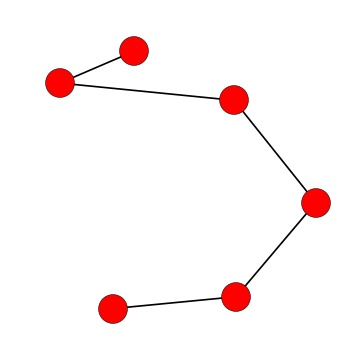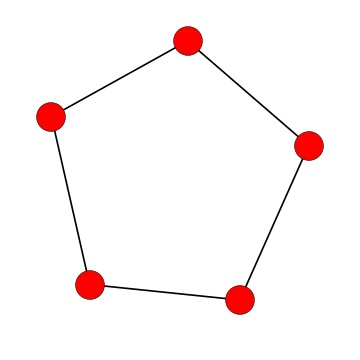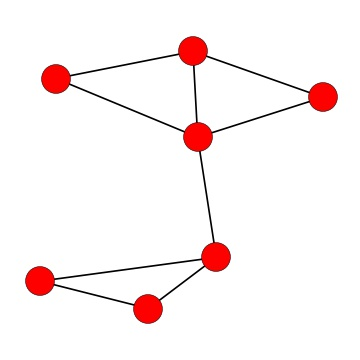Probability

# Hamiltonian PathsCan you draw a path that visits every node exactly once (i.e. a Hamiltonian path) on the graph above?

Is there a Hamiltonian path on the complete graph $K_n?$ (Note: the complete graph $K_n$ is the graph with $n$ nodes where every pair of nodes has an edge between them.)How many Hamiltonian paths are there on the graph above?Does the graph above have a Hamiltonian path?

True or false: all Hamiltonian paths are Eulerian paths. (Note: an Eulerian path is one that uses every edge.)

×